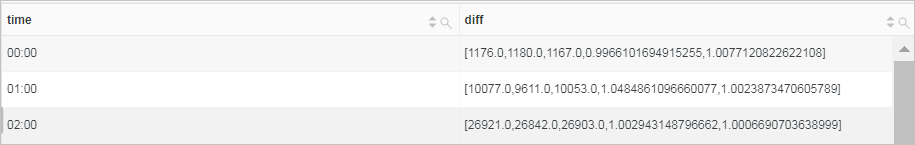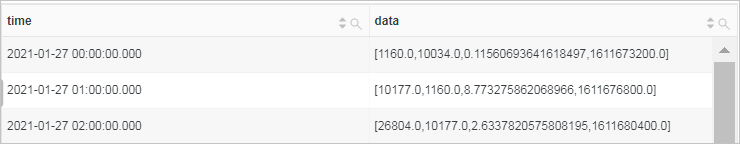This topic describes the basic syntax of interval-valued comparison and periodicity-valued comparison functions. This topic also provides related examples.

## compare() function

The compare() function is used to compare the calculation result of the current time period with the calculation result before N seconds. You can use this function to perform an interval-valued comparison or periodicity-valued comparison on data.

• Syntax
``compare(column name,N)``
Note The compare() function can be used to compare the calculation results of multiple periods of time, for example, compare(column name,N1,N2,N3).
• column name: The name of the specified column. The value of this parameter must be of the double or long type.
• N: The time window. Unit: seconds. Example: 3600 (1 hour), 86400 (one day), or 604800 (one week).
• Result

The returned result is a JSON array in the following format: [the current value, the value before N seconds, the ratio of the current value to the value before N seconds, the UNIX timestamp before N seconds]. Example: [1176.0,1180.0,0.9966101694915255,1611504000.0].

• Examples
• Calculate the ratio of the PVs of the current hour to the PVs of the same time period the day before.

Set the time range to 1 Hour(Time Frame) and execute the following query statement. 86400 indicates the current time minus 86400 seconds (one day). log indicates the Logstore name.

``* | SELECT compare(PV, 86400) FROM (SELECT count(*) AS PV FROM log)``
The following figure shows the returned result.• 3337.0 indicates the PVs of the current 1 hour, for example, Dec 25, 2020, 14:00:00 ~ Dec 25, 2020, 15:00:00.
• 3522.0 indicates the PVs of the same time period the day before, for example, Dec 24, 2020, 14:00:00 ~ Dec 24, 2020, 15:00:00.
• 0.947473026689381 indicates the ratio of the PVs of the current hour to the PVs of the same time period the day before.
To display the analysis results in multiple columns, you can execute the following query statement:
``* | SELECT diff AS today, diff AS yesterday, diff AS ratio FROM (SELECT compare(PV,86400) AS diff FROM (SELECT count(*) AS PV FROM log))``
The following figure shows the returned result.Note

To compare the data of a specified year or week with the data of the previous year or week, you can use the query statements in the following examples:

• For example, assume that you need to calculate the ratio of the PVs of November 2020 to the PVs of November 2019. You can set the time range to Nov 1, 2020, 00:00~Dec 1, 2020, 00:00, and execute the following query statement:
``* | SELECT compare(PV, 31622400) FROM (SELECT count(*) AS PV FROM log)``
• For example, assume that you need to calculate the ratio of the PVs of a Tuesday to the PVs of the previous Tuesday. You can set the time range to Jan 18, 2021, 00:00~Jan 1, 2021, 00:00, and execute the following query statement:
``* | SELECT compare(PV, 604800) FROM (SELECT count(*) AS PV FROM log)``
• Calculate the ratio of the PVs of every hour today to the PVs of the same time period the day before and two days before.

Set the time range to Today(Time Frame) and execute the following query statement. 86400 indicates the current time minus 86400 seconds (one day). 172800 indicates the current time minus 172800 seconds (two days). log indicates the Logstore name. date_format(from_unixtime(__time__), '%H:00') indicates the returned time format.

``* | SELECT time, compare(PV, 86400,172800) as diff from (SELECT count(*) as PV, date_format(from_unixtime(__time__), '%H:00') as time from log GROUP BY time) GROUP BY time ORDER BY time``
The following figure shows the returned result.• 1176.0 indicates the PVs of the current time period, for example, Dec 25, 2020, 00:00 ~ Dec 25, 2020, 01:00.
• 1180 indicates the PVs of the same time period the day before, for example, Dec 24, 2020, 00:00 ~ Dec 24, 2020, 01:00.
• 1167.0 indicates the PVs of the same time period two days before, for example, Dec 23, 2020, 00:00:00 ~ Dec 23, 2020, 01:00:00.
• 0.9966101694915255 indicates the ratio of the PVs of the current time period to the PVs of the same time period the day before.
• 1.0077120822622108 indicates the ratio of the PVs of the current period to the PVs of the same period two days before.
To display the analysis results in multiple columns, you can execute the following query statement:
``* | SELECT time, diff AS day1, diff AS day2, diff AS day3, diff AS ratio1,  diff AS ratio2 FROM (SELECT time, compare(PV, 86400,172800) as diff from (SELECT count(*) as PV, date_format(from_unixtime(__time__), '%H:00') as time from log GROUP BY time) GROUP BY time ORDER BY time)``
The following figure shows the returned result.• Calculate the ratio of the PVs of December to the PVs of November in the same year.

Set the time range to This Month(Time Frame) and execute the following query statement. 2592000 indicates the current time minus 2592000 seconds (one month). log indicates the Logstore name. date_trunc('month', __time__) indicates that the date_trunc function is used to truncate a time by month.

``*| SELECT time, compare(PV, 2592000) AS diff from (SELECT count(*) AS PV, date_trunc('month', __time__) AS time from log GROUP BY time) GROUP BY time ORDER BY time``
The following figure shows the returned result.## ts_compare() function

The ts_compare() function is used to compare the calculation result of the current time period with the calculation result before N seconds. You can use this function to perform an interval-valued comparison or periodicity-valued comparison on time series data. The analysis results of the ts_compare() function must be grouped by the time column by using the GROUP BY clause.

• Syntax
``ts_compare(column name,N)``
Note The ts_compare() function can be used to compare the calculation results of multiple periods of time, for example, ts_compare(column name,N1,N2,N3).
• column name: The name of the specified column. The value of this parameter must be of the double or long type.
• N: The time window. Unit: seconds. Example: 3600 (1 hour), 86400 (one day), or 604800 (one week).
• Result

The returned result is a JSON array in the following format: [the current value, the value before N seconds, the ratio of the current value to the value before N seconds, the UNIX timestamp before N seconds]. Example: [1176.0,1180.0,0.9966101694915255,1611504000.0].

• Example

Calculate the ratio of the PVs of every hour today to the PVs of the previous hour.

Set the time range to Today(Relative) and execute the following query statement. 3600 indicates the current time minus 3600 seconds (1 hour). log indicates the Logstore name. date_trunc('hour',__time__ ) indicates that the date_trunc function is used to truncate a time by hour.

``* | SELECT time, ts_compare(PV, 3600) AS data FROM(SELECT date_trunc('hour',__time__ ) AS time, count(*) AS PV from log GROUP BY time ORDER BY time ) GROUP BY time``
The following figure shows the returned result.